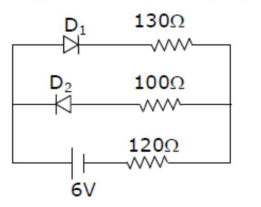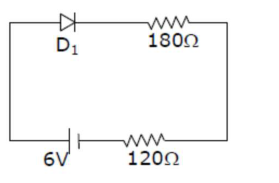# The circuit contains two diodes each with a forward resistanceQuestion:

The circuit contains two diodes each with a forward resistance of $50 \Omega$ and with infinite reverse resistance. If the battery voltage is $6 \mathrm{~V}$, the current through the $120 \Omega$ resistance is______ $\mathrm{mA}$Solution:

$(\mathbf{2 0})$

$\mathrm{D}_{2}$ is reverse bias so current does not flow through $\mathrm{D}_{2}$

$\mathrm{D}_{1}$ is forward bias.$I=\frac{6}{300} \quad \Rightarrow 0.02 \mathrm{~A}$

$=20 \mathrm{~mA}$